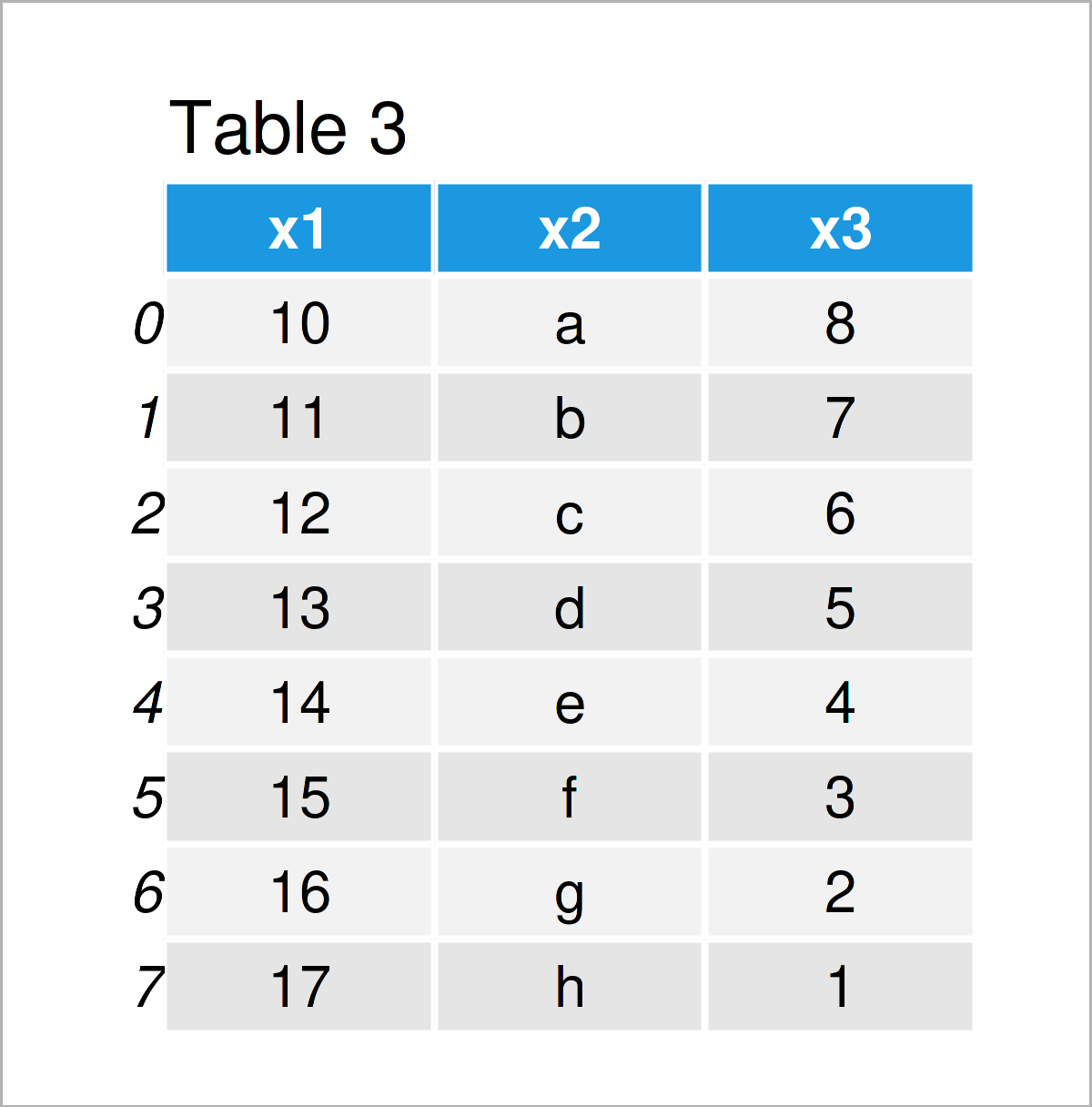# Reindex & Reset Index of pandas DataFrame from 0 in Python (3 Examples)

This tutorial shows how to reset and reindex the indices of a pandas DataFrame in the Python programming language.

Let’s dive right in!

## Example Data & Libraries

We first need to import the pandas library, if we want to use the corresponding functions:

`import pandas as pd                          # Import pandas`

Let’s also define some exemplifying data in Python:

```data = pd.DataFrame({'x1':range(10, 18),     # Create pandas DataFrame
'x2':['a', 'b', 'c', 'd', 'e', 'f', 'g', 'h'],
'x3':range(8, 0, - 1)},
index = [5, 6, 2, 1, 4, 7, 0, 3])
print(data)                                  # Print pandas DataFrame```The previous table visualizes that our example pandas DataFrame has eight rows and three columns. The indices of this DataFrame are not ordered.

## Example 1: Reindex pandas DataFrame Using reindex() Function

In this section, I’ll illustrate how to change the ordering of the indices of a pandas DataFrame in Python.

For this task, we first have to create a list that specifies the new order of our data set. Note that the values in this list are the same as the current index values:

```new_index = [1, 6, 2, 7, 0, 3, 5, 4]         # Create list for new index
print(new_index)                             # Print new index
# [1, 6, 2, 7, 0, 3, 5, 4]```

Next, we can sort the rows of our pandas DataFrame according to this list using the reindex function:

```data_new1 = data.reindex(new_index)          # Apply reindex function
print(data_new1)                             # Print updated DataFrame```As shown in Table 2, we have created a new pandas DataFrame by running the previous syntax. As you can see, the rows of this DataFrame have been rearranged according to the ordering in our list object.

## Example 2: Reset Index of pandas DataFrame from 0 Using reset_index() Function

In Example 2, I’ll show how to reset the index numbers of a pandas DataFrame from 0 to the number of rows of the DataFrame.

To achieve this, we can apply the reset_index function as illustrated in the Python syntax below.

Within the reset_index function, we are also specifying the drop argument to be equal to True. This is an optional step. If we avoid this argument, a new column containing the original index will be added to the output.

Anyway, let’s do this in practice:

```data_new2 = data.reset_index(drop = True)    # Apply reset_index function
print(data_new2)                             # Print updated DataFrame```In Table 3 you can see that we have constructed a new pandas DataFrame with indices ranging consecutively from 0 to the number of rows of our data set.

Note that the values in the rows of this new data set have not been reordered, i.e. each row has a new index.

It is also possible to rearrange the values in a pandas DataFrame together with the indices, and this is what I will show you in the next example!

## Example 3: Sort Rows of pandas DataFrame by Index Using sort_index() Function

This example shows how to order the rows of a pandas DataFrame by its index.

For this, we can use the sort_index function as shown below:

```data_new3 = data.sort_index()                # Apply sort_sindex function
print(data_new3)                             # Print updated DataFrame```As shown in Table 4, we have created another pandas DataFrame where the rows have been reordered according to the index numbers.

## Video, Further Resources & Summary

I have recently released a video on my YouTube channel, which shows the Python programming codes of this article. You can find the video below.

Please accept YouTube cookies to play this video. By accepting you will be accessing content from YouTube, a service provided by an external third party.If you accept this notice, your choice will be saved and the page will refresh.

In addition, you could have a look at the related articles on this website. Some articles are listed below:

On this page you have learned how to reset, drop, and change the index of a pandas DataFrame in the Python programming language. In case you have any further comments or questions, don’t hesitate to let me know in the comments section.

Subscribe to the Statistics Globe Newsletter